# RD Sharma Class 8 Solutions Chapter 1 Rational Numbers Ex 1.6

In this chapter, we provide RD Sharma Class 8 Solutions Chapter 1 Rational Numbers Ex 1.6 for English medium students, Which will very helpful for every student in their exams. Students can download the latest RD Sharma Class 8 Solutions Chapter 1 Rational Numbers Ex 1.6 Maths pdf, free RD Sharma Class 8 Solutions Chapter 1 Rational Numbers Ex 1.6 Maths book pdf download. Now you will get step by step solution to each question.

### RD Sharma Solutions for Class 8 Chapter 1 Rational Numbers Ex 1.6 Download PDF

Question 1.
Verify the property : x x y = y x x by taking :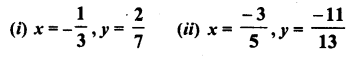Solution: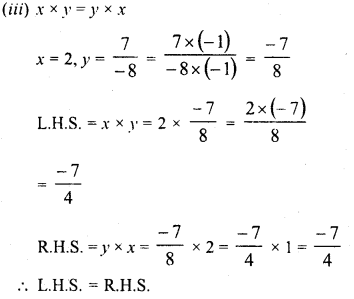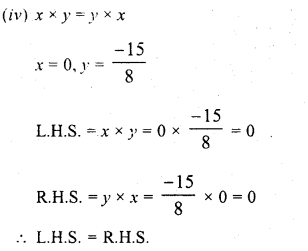Question 2.
Verify the property : x x (y x z) = (x x y) x z by taking :Solution:Question 3.
Verify the property :xx(y + 2) = xxy + x x z by taking :Solution:Question 4.
Use the distributivity of multiplication of rational numbers over their addition to simplify :Solution: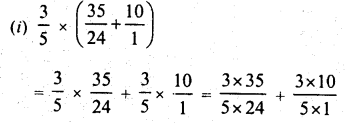Question 5.
Find the multiplicative inverse (reciprocal) of each of the following rational numbers :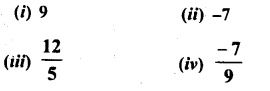Solution: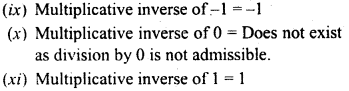Question 6.
Name the property of multiplication of rational numbers illustrated by the following statements :Solution:Question 7.
(i) The product of two positive rational numbers is always______.
(ii) The product of a positive rational number and a negative rational number is always________.
(iii) The product of two negative rational numbers is always________.
(iv) The reciprocal of a positive rational number is________.
(v) The reciprocal of a negative rational number is________.
(vi) Zero has reciprocal. The product of a rational number and its reciprocal is______.
(viii) The numbers and are their own reciprocals______.
(ix) If a is reciprocal of b, then the reciprocal of b is______.
(x) The number 0 is the reciprocal of any number______.
(xi) Reciprocal of 1a, a≠ 0 is______.
(xii) (17 x 12)-1 = 17-1 x________ .

Solution:
The product of two positive rational numbers is always positive.
(ii) The product of a positive rational number and a negative rational number is always negative.
(iii) The product of two negative rational numbers is always positive.
(iv) The reciprocal of a positive rational number is positive.
(v) The reciprocal of a negative rational number is negative.
(vi) Zero has no reciprocal.
(vii) The product of a rational number and its reciprocal is 1.
(viii)The numbers 1 and -1 are their own reciprocals.
(ix) If a is reciprocal of b, then the reciprocal of b is a.
(x) The number 0 is not the reciprocal of any number.
(xi) Reciprocal of 1a, a≠ 0 is a.
(xii) (17 x 12)-1 = 17-1 x________ .

Question 8.
Fill in the blanks :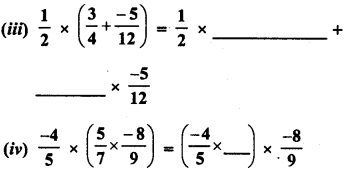Solution:
Fill in the blanks :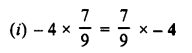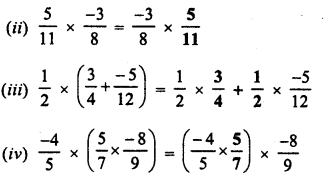All Chapter RD Sharma Solutions For Class 8 Maths

—————————————————————————–

All Subject NCERT Exemplar Problems Solutions For Class 8

All Subject NCERT Solutions For Class 8

*************************************************

I think you got complete solutions for this chapter. If You have any queries regarding this chapter, please comment on the below section our subject teacher will answer you. We tried our best to give complete solutions so you got good marks in your exam.

If these solutions have helped you, you can also share rdsharmasolutions.in to your friends.